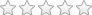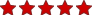You are not logged in.Language:Basic Chemistry for OperatorsThis course teaches basic chemistry skills that will enhance operator understanding and subsequent learning.  CE value = 1.25 hours.  Topics covered include specific gravity, atomic structure, number and weight, units and measurement, chemical reactions, pH, solutions and suspensions and more.  Topics are applied to water and wastewater operations in a practical manner.  NOTE: If you wish to use this course for CE, please access it in the Continuing Education catalog.

Estimated Length: 1 hour(s) and 15 minute(s)

## Lessons

Name View Schedule1. Basic Chemistry for Operators -

## Objectives

Upon completion of this course, the learner will be able to:

1. List and define the states of matter
2. Define and calculate specific gravity and density of a substance
3. Identify the common forms of energy
4. Identify and apply common units of measurement
5. Calculate unit conversions
6. Apply scientific notation
7. Define basic chemistry terms such as atom, element, compound, ion, isotope
8. Sketch and label a simple atom
9. Define and apply atomic weight, atomic number and mole
10. Read and apply chemical symbols and equations
11. Calculate formula weight
12. Define and list the properties of acids and bases
13. Identify common chemicals and their effect on pH
14. Define and apply the concept of pH
15. Define and apply solutions and the parts thereof
16. Define and apply suspensions, colloids, electrolytes, conductivity
17. Perform calculations using the two and three normal equations
18. Identify and describe basic analytical processes and quality control measures
ExpertJim Matthews